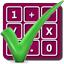#### Arithmetic of Computers

#### from Tenscope Limited

By using this site, you are accepting "session" cookies, as set out in the site's Privacy Policy
Cookies are also used to remember which page of the book you last viewed, so that when you revisit the site you automatically return to the last page you visited.

### Lesson 1

#### Page 8

Your answer :
Only .29 is written in the decimal system.
Well, let’s see.
You once learned that
.29  = (29)/(100),  and .4  = (4)/(10),  and .333  = (333)/(1000).
Fractional quantities such as .29, .4 and .333, written with the aid of the decimal point, are called decimal fractions. You probably were thinking about this use of the word “decimal” when you decided that the decimal fraction .29 is written in the decimal system and the whole number 492 is not.
The fact that no decimal point is shown does not exclude the number 492 from the decimal system. The word “decimal” means “ten”. The decimal system is a number system which uses ten different digits. But whole and fractional numbers may be written with decimal system digits.
The number 492 and the number .29 are both written in the decimal system because they both use the decimal system digits - which are 0, 1, 2, 3, 4, 5, 6, 7, 8 and 9.
Please return to page 1 and choose another answer.

Answer to Self-Test Question 1, Lesson 1 :
Yes.